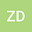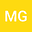loading page

A Study of Elliptic Biquaternionic Angular Momentum and Dirac Equation
••• Zülal Derin,
• Mehmet Güngör
Zülal Derin
Sakarya University Faculty of Arts and Sciences
Author ProfileMehmet Güngör
Sakarya University Faculty of Arts and Sciences
Author Profile## Abstract

In this article, we deal with the Dirac equation and angular momentum, which have an important place in physics, in terms of elliptic biquaternions. Thanks to the elliptic biquaternionic representation of angular momentum, we have expressed some useful mathematical and physical results. We have obtain the solutions of the Dirac equation with elliptic Dirac matrices. Then, we have express the elliptic biquaternionic rotational Dirac equation. This equation could be interpreted as the combination of rotational energy and angular momentum of the particle and anti particle. Therefore, we also discuss the rotational energy momentum in the Euclidean space, the elliptic biquaternionic form of the relativistic mass. Further, we expressed the spinor wave function with elliptic biquaternions. Accordingly, we also have show elliptic biquaternionic rotational Dirac energy-momentum solutions through this function.